## Change of Basis

In this page you will learn how you can transform a point from one coordinate system into another coordinate system.

In the previous topic of Basis Vector , you have learned that we can change the coordinate system. Suppose you have a point with coordinate of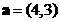in Euclidean space (with standard basis coordinate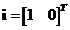and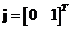). We want to find the coordinate of the same point in a new coordinate system form by basis vector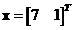and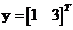. The figure below shows that the new coordinate of the same point is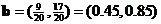. In this page, I will show you how you will obtain the coordinate in the new coordinate system.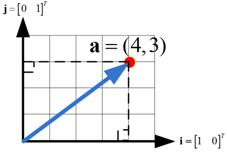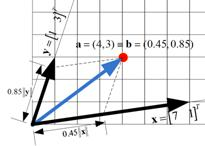Suppose we start with a point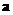in the standard Euclidean basisand. We want to transform it into a new space span by basis vectors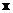and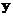. First, we can do horizontal concatenation of the new basis vectors into a matrix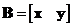. Then, the coordinate of a point in the old basisis equal to the matrix multiplication of the augmented matrix of the new basis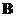with coordinate of the point in the new basis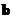. That is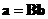. Thus to get the coordinate of a point in the new basis is the reverse, that is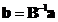.

Example:
Our point in the Euclidean basis is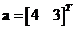. Our new basis is vectorand. Augmenting the basis vectors form a matrix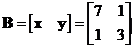. The inverse of the matrix is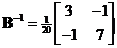. The coordinate of the point in the new coordinate is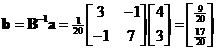.

Example:
Now suppose we want to find back the coordinate of point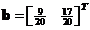from the coordinate system of basis vectorsandinto Euclidean system. Augmenting the basis vectors form a matrix. We have our coordinate point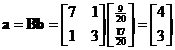back.

## Note:

Transformation of coordinate systems follows equality of matrix-vector multiplication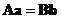, where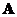andare the matrix of the respective basis vectors. In Euclidean coordinate system, the basis vectors form identity matrix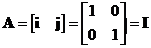. Thus, the formulacan be simplified into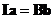or.